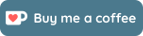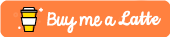# Java: Combining Postfix and Prefix Operators (a = a++ + ++a;)

Going over a lesson with my students about postfix operators vs prefix operators in Java, my students had a question about what happens when an expression has both postfix and prefix operators.

In Java, usually you would see one use ++ in a for loop. Usually the counter variable usually increases. Assuming the counter variable is i, using i++ or ++i doesn’t make a difference as seen in the examples below (both will display 12345)

```for(int i = 1; i <= 5; i++)
{
System.out.print(i);
}
```
```for(int i = 0; i <= 5; ++i)
{
System.out.print(i);
}
```

The postfix operator (for example: i++) increases the value of i by one but returns the previous value.

```int i = 0;
System.out.println(i++); // will output 0 but the value of i
// has been increased to 1

i = i++; // i will have the value of 1 since i++ increases i by 1 to 2
//but the previous value 1 is used to assign the value to i
//which makes i have a value of 1
```

The prefix operator (for example: ++i) increases the value of i by one and returns the new value.

```int i = 0;
System.out.println(++i); // will output 1 and the value of i
// has been increased to 1

i = ++i; // i will have the value of 2 as ++i increases i by 1 to 2
// the new value 2 is assigned to the value of i which makes i have a value of 2
```

However, what happens when they are used in other expressions with other operators such as:

```// Example 1
int a = 1;
a = a++ + ++a;
System.out.println(a);
```

Before we begin, it’s good to take a look at the Operator Precedence table for Java (Oracle). I’ve added in associativity based on the table found here. Operators that are higher in the table are performed before operators that are lower in the table. For instance, multiplicative operations will be performed before additive operations.

 Operators Precedence Associativity postfix expr++    expr– not associative unary ++expr   –expr  +expr  -expr    ~    ! right to left multiplicative *   /    % left to right additive +   – left to right shift <<    >>   >>> left to right relational <   >   <=   >=   instanceof not associative equality ==   != left to right bitwise AND & left to right bitwise exclusive OR ^ left to right bitwise inclusive OR | left to right logical AND && left to right logical OR || left to right ternary ?  : right to left assignment =  +=   -=   *=   /=   %=   ^=  |=   <<=   >>=   >>>= right to left

Before Java evaluates an expression, it must first determine the value of any of the variables used in the expression. It does this according to the associativity of the operators.

So in example 1, it first scans through the expression filling out the values of a from left to right as + goes from left to right on the right side of the assignment operator (=) which is performed last due to it’s precedence.

```// Example 1
int a = 1;
a = a++ + ++a;
//  1   +   3
System.out.println(a);
```

Since a starts at 1, when it reaches a++, a increases to 2 but the old value of 1 is returned. When it reaches ++a, since a already increased by 1 from a++, ++a increases a to 3 and uses the new value, which is 3. Thus a = a++ + ++a is evaluated as a = 1 + 3 which gives us 4.

Let’s look at another example

```// Example 2
int a = 2;
a = ++a * a++;
//    3 * 3
System.out.println(a);
```

In Example 2, again we fill in the values of a on the right side of = before we assign the value back to a. When ++a is reached, it it increases a to 3 and uses that new value. When a++ is reached, it a increases to 4 but uses the old value of 3. Thus a is assigned the value of 9.

In Example 3, we switch the order found in Example 2.

```// Example 3
int a = 2;
a = a++ * ++a;
//  2   *   4
System.out.println(a);
```

In example 3, a++ increases a to 3, but uses the old value 2. When we get to ++a, value of a is 3 so it increases to 4 and we use the new value. Thus, a is assigned the value of 8.

One important thing to note is that precedence is not taken into consideration when filling in the values of variables. The precedence takes in effect once all the values are known.

Example 4 will show the difference.

```// Example 4
int a = 1;
a = a++ + ++a * a;
//  1   +   3 * 3
System.out.println(a);

int b = 1;
b = b++ * ++b + b;
//  1   *   3 + 3
System.out.println(b);
```

In example 4, the operations are the same, but the order is switched. For variable a, a++ increases a to 2 but the old value of 1 is used. ++a increases a to the value of 3 and the new value, 3 is used. Variable a at the end is now 3, so a gets assigned the value of 1 + 3*3 which is 10.

Variable b has the same values as variable a. However, since the operation orders are different, b gets assigned the value 1 * 3 + 3 which is 6.

To end this post, we will do one more example

```// Example 5
int a = 2;
a = a + a++ - a-- * --a + ++a + a
//  2 + 2   - 3   *   1 +   2 + 2
System.out.println(a);
```

In example 5, a starts as 2 when we reach a++, a increases to 3 but the old value is used which is 2. At a– the value of a is 3 but gets decreased to 2 and the old value, 3, is used. When it gets to –a, a is decreased to 1 and we use this new value. At ++a, a is increased to 2 and we use this value. At the last a, a is now 2, so it’s value is 2. Finally, a is assigned the value 2 + 2 – 3 * 1 + 2 + 2 which gives us the value of 5.

So as you can see, using multiple postfix and prefix increment operators in one expression is confusing. So no one really does this in real life. Always remember to keep your code clear so other people can understand and maintain your code.

## Support

If you like my work, a small donation or feedback will be much appreciated.